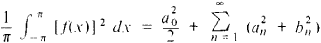# Parseval Equality

## Parseval Equality

an equation of the formwhere a0 an, and bn are the Fourier coefficients for f(x). It was established in 1805 by the French mathematician M. Parseval by assuming that trigonometric series could be termwise integrated. In 1896, A. M. Liapunov showed that the equality is valid if the function is bounded on the interval (—π, π) and if the integral ∫πf(x) dx exists. It was later proved that the Parseval equality holds for any function whose square is integrable. V. A. Steklov demonstrated that the Parseval equality is valid for series in other orthogonal systems of functions.

References in periodicals archive ?
By the expansions (11) and (14), using Parseval equality, we obtain that
and applying the Parseval equality [MATHEMATICAL EXPRESSION NOT REPRODUCIBLE IN ASCII], we obtain
Using the last theorem and Parseval equality, we obtain
By the Parseval equality we have [MATHEMATICAL EXPRESSION NOT REPRODUCIBLE IN ASCII].
[K.sub.d] ([psi], [chi]) can be evaluated either by direct integration or using convolution formula and Parseval equality for harmonic analysis on [S.sub.d-1].
By Parseval equality for harmonic analysis on [S.sub.d-1] we obtain the following expression:
Since the spectrum is simple and discrete, then Parseval equality (6) yields for any sequence of values F([[lambda].sub.n]) such that
Then the Fourier coefficients of the difference [f.sub.1] - f are zero and applying the Parseval equality (3.9) to the function [f.sub.1] - f we get that [f.sub.1] - f = 0, so that the sum of series (4.1) is equal to f(t).
and that f (b) = 0 in case b is left-scattered, we have the Parseval equality (see )
We will show that the Parseval equality for problem (1.1), (1.2) can be obtained from (3.4) by letting b [right arrow] [infinity].
Now we apply Parseval equality (3.4) to the function (assuming b > [t.sub.0])

Site: Follow: Share:
Open / Close